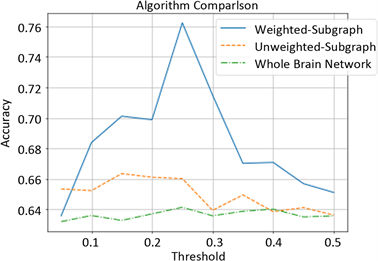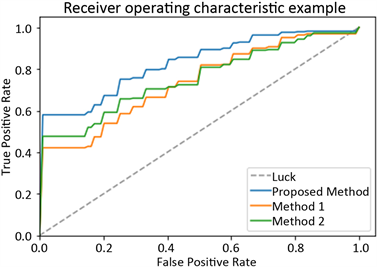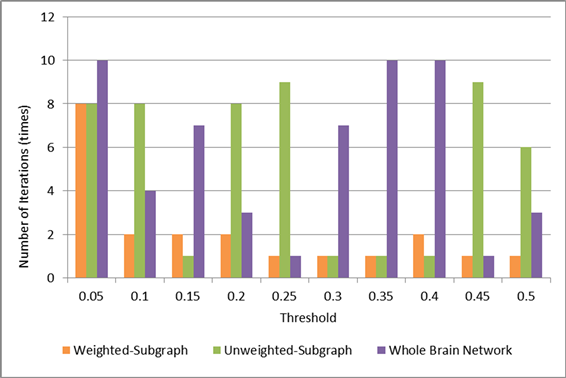# 基于最短路径的关键子网选择的加权脑网络分类研究Research on Classification of Weighted Brain Network Based on Key Subnet Selection of Shortest Path

DOI: 10.12677/CSA.2020.109165, PDF, HTML, XML, 下载: 110  浏览: 328

Abstract: At present, most of classifications of mild cognitive impairment are based on the local correlation of a single brain region in the whole brain network, which ignores the information of interaction between multiple brain regions. Moreover, most of the current researches are based on the unweighted brain network, losing the intensity of interaction between brain regions. In order to better capture the key topology information that can distinguish brain networks, this paper proposes a key subnet selection algorithm. Firstly, the shortest path in weighted brain network is calculated, and features with significant difference are selected according to the difference of the number of the shortest path in positive and negative samples. Then, the key subnets are constructed according to the nodes on the selected shortest paths, and finally graph kernel is used to classify subnet datasets. By comparing the weighted brain network, the unweighted brain network that implement the pro-posed algorithm and the whole brain network, the effectiveness of this method in the classification of mild cognitive impairment is verified.

1. 引言

2. 相关技术

2.1. 皮尔逊相关系数(Pearson Correlation Coefficient)

$r=\frac{\sum \left(x-\stackrel{¯}{x}\right)\left(y-\stackrel{¯}{y}\right)}{\sqrt{\sum {\left(x-\stackrel{¯}{x}\right)}^{2}\sum {\left(y-\stackrel{¯}{y}\right)}^{2}}}$ (1)

2.2. 最短路径

2.3. 图核

3. 关键子网选择算法的提出

3.1. 关键子网选择算法简介

3.2. 关键子网选择算法研究

MCI患者脑网络中最短路径上的节点及条数与正常人相比会有明显差异，因而，存在某一最短路径在所有正类样本中出现的次数多于或者少于在所有负类样本中出现的次数，为此对度量某一最短路径在样本集合中出现的次数做了如式(2)的定义。

${f}_{q}\left({l}_{s}|L\right)=\frac{|{l}_{s}|}{|L|}$ (2)

$S\left({l}_{s}\right)=|{f}_{q}\left({l}_{s}|{L}_{p}\right)-{f}_{q}\left({l}_{s}|{L}_{n}\right)|$ (3)

$S\left({l}_{p}^{1}\right)\ge S\left({l}_{p}^{2}\right)\ge \cdots \ge S\left({l}_{p}^{m}\right)$ (4)

$S\left({l}_{n}^{1}\right)\ge S\left({l}_{n}^{2}\right)\ge \cdots \ge S\left({l}_{n}^{k}\right)$ (5)

$J\left(R\right)=\underset{|\left\{{l}_{p}^{i}|i\le m\right\}|\le {r}_{1}}{\sum }S\left({l}_{p}^{i}\right)+\underset{|\left\{{l}_{n}^{j}|j\le k\right\}|\le {r}_{2}}{\sum }S\left({l}_{n}^{j}\right)$ (6)

①对正类和负类样本组分别计算其脑网络中的所有最短路径，取并集后分别得到候选的最短路径集合 ${L}_{p}$${L}_{n}$

②对 ${L}_{p}$${L}_{n}$ 中的每一条最短路径，分别利用式(3)计算其判别性得分并按照降序排列，如式(4)和(5)所示；

③根据式(6)选择出式(4)和(5)中判别性得分最高的前 ${r}_{1}$${r}_{2}$ 条最短路径，可得到最优特征子集 ${R}^{*}=\left\{{l}_{p}^{i},{l}_{n}^{j}|i\le {r}_{1},j\le {r}_{2}\right\}$

④找出最优特征子集 ${R}^{*}$ 上的所有节点，取并集并构建所有训练样本的关键子网，得到 ${G}_{train}=\left\{{G}_{1},{G}_{2},\cdots ,{G}_{N}\right\}$

4. 实验及结果分析

4.1. 数据集Table 1. Sample information details

4.2. 脑网络的构建

${G}^{\prime }=\left\{\begin{array}{l}0,\text{\hspace{0.17em}}\text{\hspace{0.17em}}\text{\hspace{0.17em}}\text{\hspace{0.17em}}\text{\hspace{0.17em}}\text{\hspace{0.17em}}\text{\hspace{0.17em}}\text{\hspace{0.17em}}\text{\hspace{0.17em}}\text{\hspace{0.17em}}g\left(i,j\right) (7)

4.3. 实验结果与分析

4.3.1. 分类效果对比分析Table 2. Comparison of classification effects of different brain networksFigure 1. Classification effects of different brain networks under different thresholdsFigure 2. Average ROC curve of different brain networks

4.3.2. Weisfeiler-Lehman子树核迭代次数对比

Weisfeiler-Lehman子树核的迭代次数在一定程度上反映了两组被试者脑网络的拓扑结构是否存在较为明显的差异。若该图核的迭代次数越多，则表明MCI患者和正常人脑网络的结构差异并不明显，需要较多次数的迭代才能将两者区分开；反之，较少的迭代次数表明两组被试者脑网络的结构差异较为明显，仅需要较少的迭代次数便可区分两者。因而，实验记录了不同阈值下的加权子网络、无权子网络及全脑网络达到最高分类准确率时，Weisfeiler-Lehman子树核所需要的迭代次数，具体信息如图3所示。Figure 3. The number of iterations of different brain networks under different thresholdsTable 3. Statistics of iteration times of different brain networks

5. 结束语

  Petersen, R.C., Doody, R., Kurz, A., et al. (2001) Current Concepts in Mild Cognitive Impairment. Archives of Neurolo-gy, 58, 1985-1992. https://doi.org/10.1001/archneur.58.12.1985  Petersen, R.C., Smith, G.E., Waring, S.C., et al. (1999) Mild Cognitive Impairment: Clinical Characterization and Outcome. Archives of Neurology, 56, 303-308. https://doi.org/10.1001/archneur.56.3.303  Brier, M.R., Thomas, J.B., Snyder, A.Z., et al. (2012) Loss of In-tranetwork and Internetwork Resting State Functional Connections with Alzheimer’s Disease Progression. The Journal of Neuroscience: The Official Journal of the Society for Neuroscience, 32, 8890-8899. https://doi.org/10.1523/JNEUROSCI.5698-11.2012  胡瑞红, 范存秀, 毕晓莹. 轻度认知功能障碍的神经影像学最新研究进展[J]. 中国卒中杂志, 2019, 14(3): 297-300.  Yuan, W., He, K., Guan, D., et al. (2019) Graph Kernel Based Link Prediction for Signed Social Networks. Information Fusion, 46, 1-10. https://doi.org/10.1016/j.inffus.2018.04.004  Wang, W., Jie, B., Bian, W., et al. (2019) Graph-Kernel Based Structured Feature Selection for Brain Disease Classification Using Functional Connectivity Networks. IEEE Access, 7, 35001-35011. https://doi.org/10.1109/ACCESS.2019.2903332  Gallo, G. and Pallottino, S. (1988) Shortest Path Algorithms. Annals of Operations Research, 13, 1-79. https://doi.org/10.1007/BF02288320  乐阳, 龚健雅. Dijkstra最短路径算法的一种高效率实现[J]. 武汉测绘科技大学学报, 1999(3): 3-5.  白璐, 徐立祥, 崔丽欣, 等. 图核函数研究现状与进展[J]. 安徽大学学报(自然科学版), 2017, 41(1): 21-28.  Vishwanathan, S.V.N., Schraudolph, N.N., Kondor, R., et al. (2010) Graph Ker-nels. Journal of Machine Learning Research, 11, 1201-1242.  Shervashidze, N., Schweitzer, P., Jan, E., et al. (2011) Weisfeiler-Lehman Graph Kernels. The Journal of Machine Learning Research, 12, 2539-2561.  Verberk, I.M.W., Slot, R.E., Verfaillie, S.C., et al. (2018) Plasma Amyloid as Pre-Screener for the Earliest Alzheimer Pathological Changes. Annals of Neurology, 84, 648-658. https://doi.org/10.1002/ana.25334  Troyer, A.K., Murphy, K.J., Anderson, N.D., et al. (2012) Associative Recognition in Mild Cognitive Impairment: Relationship to Hippocampal Volume and Apolipoprotein E. Neuropsychologia, 50, 3721-3728. https://doi.org/10.1016/j.neuropsychologia.2012.10.018  Tzourio-Mazoyer, N., Landeau, B., Papathanassiou, D., et al. (2002) Automated Anatomical Labeling of Activations in SPM Using a Macroscopic Anatomical Parcellation of the MNI MRI Single-Subject Brain. Neuroimage, 15, 273-289. https://doi.org/10.1006/nimg.2001.0978  Zanin, M., Sousa, P., Papo, D., et al. (2012) Optimizing Functional Network Representation of Multivariate Time Series. Scientific Reports, 2, Article No. 630. https://doi.org/10.1038/srep00630